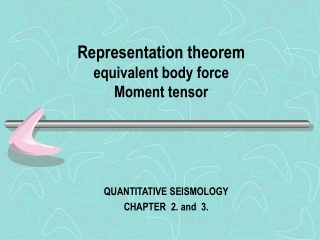DownloadDownload PresentationRepresentation theorem equivalent body force Moment tensor

# Representation theorem equivalent body force Moment tensor

Télécharger la présentation## Representation theorem equivalent body force Moment tensor

- - - - - - - - - - - - - - - - - - - - - - - - - - - E N D - - - - - - - - - - - - - - - - - - - - - - - - - - -
##### Presentation Transcript

1. Representation theoremequivalent body forceMoment tensor QUANTITATIVE SEISMOLOGY CHAPTER 2. and 3.

2. Reciprocity theorems: Bettis theorem

3. Bettis theorem • no initial conditions involved for u or v • It remains true if u, utt, T(u,n), f are evaluated at t1 but v, vtt, T(v,n), g are evaluated at a different time t2. • Choose t1=t and t2=τ-t and integrate from 0 to τ acceleration terms reduced to terms that depend only on the initial and final values.

4. For u and v with a quiescent past

5. Introducing Green function … ith component displacement at (x,t) due to an nth unit impulse force acting at x=ξ and t=ι

6. Bettis theorem

7. Representation theorem Interchange space and time symbols

8. Representation theorem

9. Representation theorems for an internal surface; body-force equivalents for discontinuities in Traction and displacement S Σ+ Σ- v

10. (Spatial reciprocity of G is applied) S Σ+ Σ- Σ++Σ-+S Assume both G and u satisfy homogeneous boundary conditions on S  S itself is no longer our concern

11. body-force equivalents What are body-force equivalents mean ?

12. Traction discontinuity contributes to displacement field (Delta function is used to “localized” T on Σ within V)

13. body-force equivalent of a traction discontinuity on Σ

14. displacement discontinuity contributes to displacement How to localize [u] on Σ within V ?

15. body-force equivalent of a displacement discontinuity on Σ The body-force equivalents hold for a general inhomogeneous anisotropic medium, and they are remarkable in their dependence on properties of the elastic medium only at the fault surface itself.

16. ξ3 Slip jump as a buried faulting [u]=uΣ+ - uΣ- ξ2 ξ1 Σ • Σ lies in the ξ3plane • Isotropic media • Let ξ1 the direction of slip

17. In isotropic medium, other C13pq vanish, except C1313=C1331=μ Point forces distributed over the plane η3=0- and η3=0-

18. Total moment about the η2-axis due to f1.

19. Total moment about the η2-axis due to f1.

20. Similarly, we may find the moment due to f3 • total moment from f1 and f3 =0 • body-force equivalents from slip discontinuity across the fault plane  double couple

21. η3 η3 -δ(η3) η1 η1 What does this mean ? See figure 3.3 for the distribution of body-force over the Σ plane

22. Take a Break

23. CHAPTER 3. P.46,48

24. CHAPTER 3. P.48,49,51

25. CHAPTER 3. P.51

26. Chapter 2. P.27,28